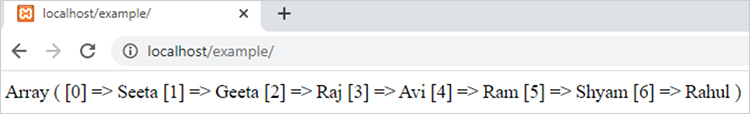array_merge() function in php || PHP Functions# array_merge() function in php

1799

array_merge function of PHP is used to merge the items of two arrays. It combines the arrays in a way that the values of second array is appended into the end of first array.

It is an inbuilt function of PHP.

### array_merge function syntax:

`array_merge (arr1, arr2, ...,arrN);`

Here

arr1, arr2 and arrN are the arrays which we want to merge.

Example 1:

```<?php
\$arr_1=array("Seeta","Geeta","Raj","Avi");
\$arr_2=array("Ram","Shyam","Rahul");
\$new_arr=array_merge(\$arr_1,\$arr_2);
print_r(\$new_arr);
?>```

Output:Example 2:

```<?php
\$arr_1=array("121"=>"Seeta","122"=>"Ram");
\$arr_2=array("1331"=>"Raja","1332"=>"Sattu");
\$new_arr=array_merge(\$arr_1,\$arr_2);
print_r(\$new_arr);
?>```

Output:Remark: If we apply array_merge function on different type arrays means one array is indexed and other is associative then the type of resultant array must be indexed.

Example:

```<?php
\$arr_1=array("121"=>"Seeta","122"=>"Ram");  // Associative array
\$arr_2=array("Raja","Sattu");  // Indexed array
\$new_arr=array_merge(\$arr_1,\$arr_2);   // It will be an indexed array
print_r(\$new_arr);
?>```

Output:Share:## Big Hosting Days

Upto 75@ OFF on Web Hosting

## Get a .COM for just \$6.98

Secure Domain for a Mini Price